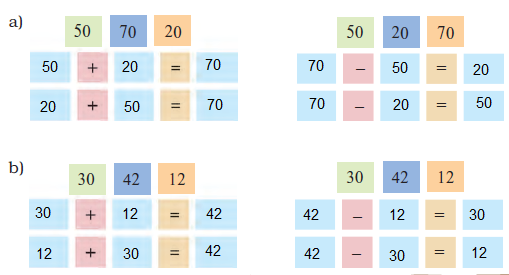-->

# STD 3 MATHS UNIT 3 GIVE AND TAKE PRACTICE TEST 1 NCERT Solutions For Class 3 Maths Chapter 3 Give and Take

### Maths NCERT Solutions for Class 3 Maths Chapter 3 Give and Take

Question 1:

Fill in the blanks

A jump from 2 to 12 is like taking 1 step.

Question 2:

How many steps did I go in all?

21 steps overall.

FIND MY FOOD

Question 1:

Fill in the blanks

a) 10 less than 34 is 24.

b) 53 – 20 = 33

c) 11 more than 31 is 42.

d) 11 less than 66 is 55.

e) 62 + 13 = 75

f) 23 less than 89 is 66.

g) 10 and 40 more is 50.

h) 9 added to 28 gives 37.

i) The sum of 9 and 44 is 53.

j) Reducing 98 by 34 gives 64.

k) 4 and 37 more is 41.

l) Take 35 away from 83. We get 48.

Question 2:

Fill in the blanks

37 + 9 = 46

65 – 30 = 35

67 = 46 + 21

57 = 87 – 30

66 – 55 = 11

45 + 54 = 99

49 + 26 = 75

56 – 21 = 35

36 = 27 + 9

40 + 36 = 76

98 = 48 + 50

57 – 37 = 20

Question 1:

See if you can do the same with these sums.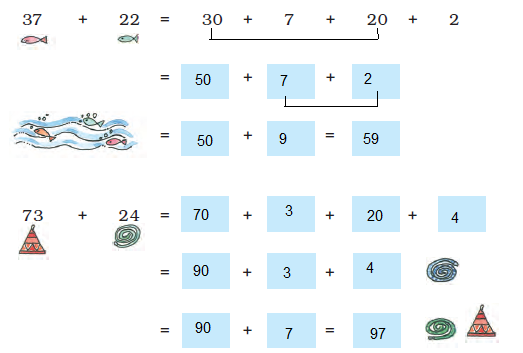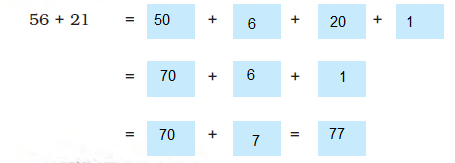LET ME TELL YOU A STORY……….

Question 1:

Fill in the blanks

33 + 42 = 75

19 + 61 = 80

90 = 48 + 42

60 = 33 + 27

97 = 34 + 63

117 = 53 + 64

55 + 25 = 80

67 + 25 = 92

72 + 56 = 128

Question 2:

The old deer said — we were 240 in number, now how many are we in all?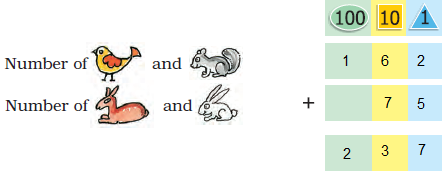Question 3:

Can you guess now, if the baby lion will go back home? Has the baby lion eaten up any animal?

No, the baby lion can not go home now. There were 240 animals but now there are only 237 of them. The baby lion has eaten 240 – 237 = 3 animals.

HOW MANY BULBS?

Question 1:

A shopkeeper Rafi had 153 candles. Paras gave him 237 more candles. How many candles does Rafi have now?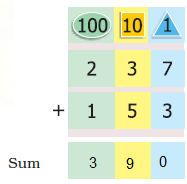Question 2:

Is the sum more than 400 or less than 400?

No, the sum is less than 400.

Question A:

A train compartment is carrying 132 people. Another compartment is carrying 129 people. In all, how many people are there in both the compartments?

Number of people in first compartment= 132

Number of people in second compartment= 129

1 3 2

+ 1 2 9

________

2 6 1 people are there in total.

Question B:

Shanu found 138 pebbles. Karim found 44 pebbles. How many pebbles did they find in all?

1 3 8

+ 4 4

________

1 8 2 pebbles in total.

Question C:

A teacher kept a note of which fruits students like in her school. This is what she found: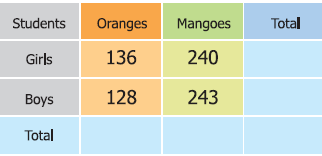Find out:

(a) How many students in the school like oranges?

(b) How many students in the school like mangoes?

(c) Altogether, how many students are there in the school?

(d) Is the number of girls more than 350 or less than 350?

(a) Number of students who like oranges = 136 + 128 = 264

(b) Number of students who like mangoes = 240 + 243 = 483

(c) Total number of students in the school = Total number of girls + Total number of boys

= (136 + 240) + (128 + 243)

= 376 + 371

= 747

(d) Number of girls = 136 + 240 = 376

Thus, the number of girls is more than 350.

PRACTICE TIME

Question A:

(i) 345 + 52 (iv) 643 + 345

(ii) 492 + 29 (v) 750 + 219

(iii) 245 + 93

(i) 397

(ii) 521

(iii) 338

(iv) 988

(v) 969

Question B:

Fill in the blanks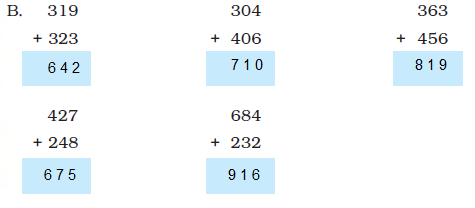Question:

Fill in the blanks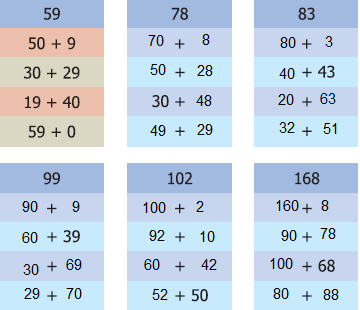Question:

Can you solve this puzzle?

Write the numbers 1, 2, 3, 4, 5, 6 in the circles, so that the sum of the numbers on each side of the figure is 12.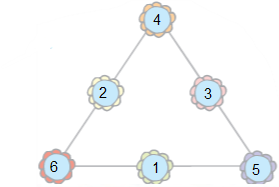Question:

Do the sums mentally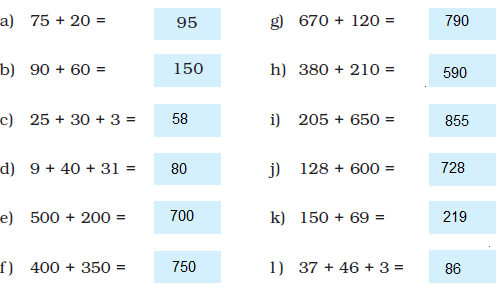Question:

Draw a line through the numbers which are answers written in the boxes above.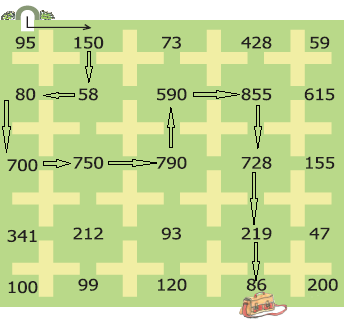CARD GAME

Question:

Here are the cards for you. Work out the combination. Place the cards in the right boxes.